# Using the functions of the ExPanDaR package

#### 2020-12-06

While the main purpose of the ExPanDaR package is to provide the infrastructure for the ExPanD app, the auxiliary functions of the package can also be used to support your exploratory data analysis workflow in your own code. All functions are relatively thin wrappers around established R packages for graphics and HTML table presentation (ggplot2, kableExtra, stargazer). While I developed them to support analysis steps that are common with empirical archival research projects in the area of accounting and finance (which happens to be my field), I hope that they are generally useful for exploratory data analysis.

To see what ExPanDaR has to offer, let’s take a quick tour. For more detailed guidance on how to use a specific function presented below, take a look at the respective function’s help page.

## Data Preparation

ExPanDaR is designed for exploratory panel data analysis (hence the name). Thus, while you can also use some functions on cross-sectional data, for most functions you will need a data frame containing your panel data. ExPanDaR expects the data to be organized in long format. This implies that each observation (a row) is identified by cross-sectional and time series identifiers and that variables are organized by columns. While you can have a vector of variables jointly determining the cross-section, the time-series needs to be identified by a unique variable. The ExPanDaR functions treat cross-sectional identifiers as factors and expect the time-series identifier to be coercible into an ordered factor.

For this walk-through I will use the data set russell_3000, which comes with the package. It contains some financial reporting and stock return data of Russell 3000 firms from Google Finance and Yahoo Finance and has been collected using the tidyquant package in the summer of 2017. A word of caution: While the data appears to be relatively decent quality I would advise against using this data for scientific work without verifying its integrity first. These are the variables included in the data.

kable(data.frame(Variable=russell_3000_data_def$var_name, Definition=sub('$', '\\$', russell_3000_data_def$var_def, fixed = TRUE)),
row.names = FALSE)
Variable Definition
coid Company identifier
period Fiscal year
coname Company name
sector Sector
industry Industry
toas Total assets at period end (M US-$) sales Sales of the period (M US-$)
equity Total equity at period end (M US-$) debt_ta Total debt (% total assets) eq_ta Total equity (% total assets) gw_ta Goodwill (% total assets) oint_ta Other intangible assets (% total assets) ppe_ta Property, plant and equipment (% total assets) ca_ta Current assets (% total assets) cash_ta Cash (% total assets) roe Return on equity (net income divided by average equity) roa Return on assets (earnings before interest and taxes divided by average equity) nioa Net income divided by average assets cfoa Cash flow from operations divided by average assets accoa Total accruals divided by average assets cogs_sales Cost of goods sold divided by sales ebit_sales Earnings before interest and taxes divided by sales ni_sales Net income divided by sales return Stock return of the period (%) You can infer from the variable definition that coid seems to identify the cross-section (a Russell 3000 firm) while period identifies the time-series (a fiscal year). In addition, coname also sounds like it mighty identify a firm but we cannot be sure whether there are duplicate company names. In addition, we want to verify that there are no duplicate coid/period pairs. Let’s check. cs_ids <- unique(russell_3000[,c("coid", "coname")]) identical(cs_ids$coid, unique(russell_3000$coid)) ##  TRUE identical(cs_ids$coname, unique(russell_3000$coname)) ##  TRUE The first test verifies that there are no two observations that share the same coid but a different coname. The second makes sure that there are firms with the same coname but a different coid. Thus, we can use both, coname and coid, or either as cross-sectional identifier. The following test establishes whether in combination coid and period identify a panel observation. any(duplicated(russell_3000[,c("coid", "period")])) ##  FALSE This seems to be the case. As a next step, let’s use ExPanDaR’s function prepare_missing_values_graph() to eyeball how frequently observations are missing in the data set. prepare_missing_values_graph(russell_3000, ts_id = "period")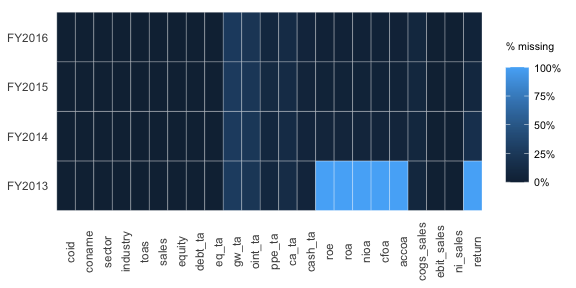OK. This does not look too bad. Only FY2013 seems odd, as some variables are completely missing. Guess why? They are calculated using lagged values of total assets. So, in the following, let’s focus on the variables that we care about and on the fiscal years 2014 to 2016 (a short panel, I know). Time to check the descriptive statistics using the prepare_descriptive_table() function. r3 <- droplevels(russell_3000[russell_3000$period > "FY2013",
c("coid", "coname", "period", "sector", "toas",
"nioa", "cfoa", "accoa", "return")])
t <- prepare_descriptive_table(r3)
t$kable_ret %>% kable_styling("condensed", full_width = F, position = "center") Descriptive Statistics N Mean Std. dev. Min. 25 % Median 75 % Max. toas 6,643 8,722.510 35,846.713 0.800 463.225 1,600.050 5,100.500 861,395.000 nioa 6,399 0.001 0.096 -2.692 -0.002 0.017 0.037 0.463 cfoa 6,399 0.033 0.084 -2.121 0.021 0.041 0.065 0.460 accoa 6,399 -0.032 0.052 -0.712 -0.046 -0.026 -0.012 0.621 return 6,009 0.097 0.433 -0.938 -0.136 0.065 0.269 6.346 Take a look at the minima and the maxima of some of the variables (e.g., net income over assets (nioa)). Normally, it should be around -50 % to + 50%. Our measure has a minimum way below -50 %. One thing that comes very handy when dealing with outliers is a quick way to observe extreme values. prepare_ext_obs_table() might be helpful here. t <- prepare_ext_obs_table(na.omit(r3[c("coname", "period", "nioa")])) t$kable_ret %>%
kable_styling("condensed", full_width = F, position = "center")
coname period nioa
Gamco Investors, Inc. FY2016 0.463
Gaia, Inc. FY2016 0.369
Five Prime Therapeutics, Inc. FY2015 0.355
NewLink Genetics Corporation FY2014 0.318
Ligand Pharmaceuticals Incorporated FY2015 0.302
Proteostasis Therapeutics, Inc. FY2015 -0.822
Proteostasis Therapeutics, Inc. FY2014 -0.830
Omeros Corporation FY2015 -1.255
vTv Therapeutics Inc. FY2014 -1.269
Omeros Corporation FY2014 -2.692

In a real life research situation, you might want to take a break and check your data as well as the actual financial statements to see what is going on. In most cases, you will see that the outliers are caused by very small denominators (average total assets in this case). To reduce the effect of these outliers on your analysis, you can winsorize (or truncate) them by using the treat_outliers() function.

r3win <- treat_outliers(r3, percentile = 0.01)
t <- prepare_ext_obs_table(na.omit(r3win[c("coname", "period", "nioa")]))
t$kable_ret %>% kable_styling("condensed", full_width = F, position = "center") coname period nioa ABIOMED, Inc. FY2015 0.147 Acacia Communications, Inc. FY2015 0.147 Acacia Communications, Inc. FY2016 0.147 Aspen Technology, Inc. FY2015 0.147 Aspen Technology, Inc. FY2016 0.147 Workhorse Group, Inc. FY2015 -0.355 Workhorse Group, Inc. FY2016 -0.355 EXCO Resources NL FY2015 -0.355 ZIOPHARM Oncology Inc FY2015 -0.355 ZIOPHARM Oncology Inc FY2016 -0.355 ## Descriptive Statistics This looks better. Let’s look at the winsorized descriptive statistics. t <- prepare_descriptive_table(r3win) t$kable_ret  %>%
kable_styling("condensed", full_width = F, position = "center")
Descriptive Statistics
N Mean Std. dev. Min. 25 % Median 75 % Max.
toas 6,643 7,198.735 17,632.076 45.400 463.225 1,600.050 5,100.500 122,418.180
nioa 6,399 0.002 0.077 -0.355 -0.002 0.017 0.037 0.147
cfoa 6,399 0.034 0.068 -0.280 0.021 0.041 0.065 0.178
accoa 6,399 -0.032 0.043 -0.209 -0.046 -0.026 -0.012 0.092
return 6,009 0.088 0.375 -0.707 -0.136 0.065 0.269 1.568

I am sure that you won’t care but I am a big fan of correlation tables. prepare_correlation_table() prepares a table reporting Pearson correlations above and Spearman correlations below the diagonal.

t<- prepare_correlation_table(r3win, bold = 0.01, format="html")
t$kable_ret %>% kable_styling("condensed", full_width = F, position = "center") A B C D E A: toas 0.10 0.07 0.08 -0.00 B: nioa 0.17 0.80 0.49 0.15 C: cfoa 0.10 0.66 -0.09 0.12 D: accoa 0.20 0.38 -0.30 0.07 E: return 0.04 0.20 0.14 0.08 This table reports Pearson correlations above and Spearman correlations below the diagonal. The number of observations ranges from 6002 to 6643. Correlations with significance levels below 1% appear in bold print. In fact, I like correlations so much that especially for samples containing many variables I use prepare_correlation_graph() to display a graphic variant based on the corrplot package. See for yourself. ret <- prepare_correlation_graph(r3win)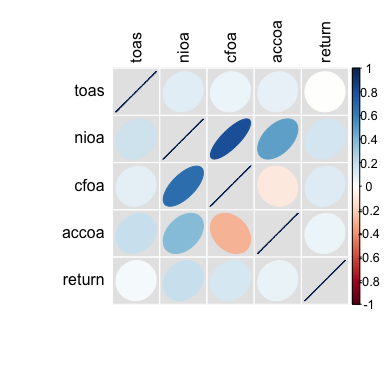## Visuals Additional visuals are available for exploring time trends. prepare_trend_graph() can be used for comparing variables… graph <- prepare_trend_graph(r3win[c("period", "nioa", "cfoa", "accoa")], "period") graph$plot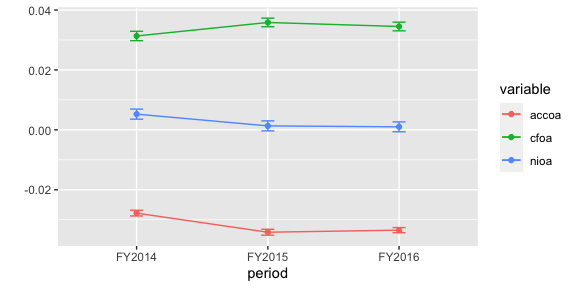… and for eyeballing the distributional properties of a single variable over time you have prepare_quantile_trend_graph().

graph <- prepare_quantile_trend_graph(r3win[c("period", "return")], "period", c(0.05, 0.25, 0.5, 0.75, 0.95))
graph$plot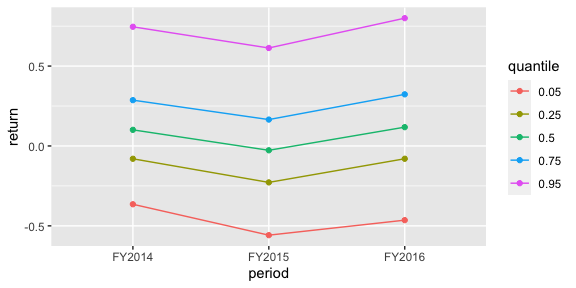Nothing special going on here (not really surprising, given the short time span that the sample covers). Let’s see how profitability varies across sectors by using the prepare_by_group_trend_graph() function. graph <- prepare_by_group_trend_graph(r3win, "period", "sector", "nioa") graph$plot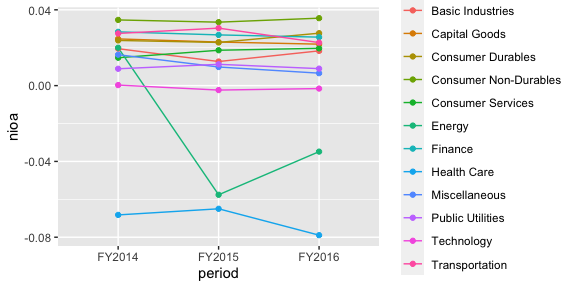The health sector is clearly less profitable compared to the others, which can be explained by small growing firms. Finally, prepare_scatter_plot() produces the mother of all plots, the scatter plot.

prepare_scatter_plot(r3win, x="nioa", y="return", color="sector", size="toas", loess = 1)
## geom_smooth() using method = 'gam' and formula 'y ~ s(x, bs = "cs")'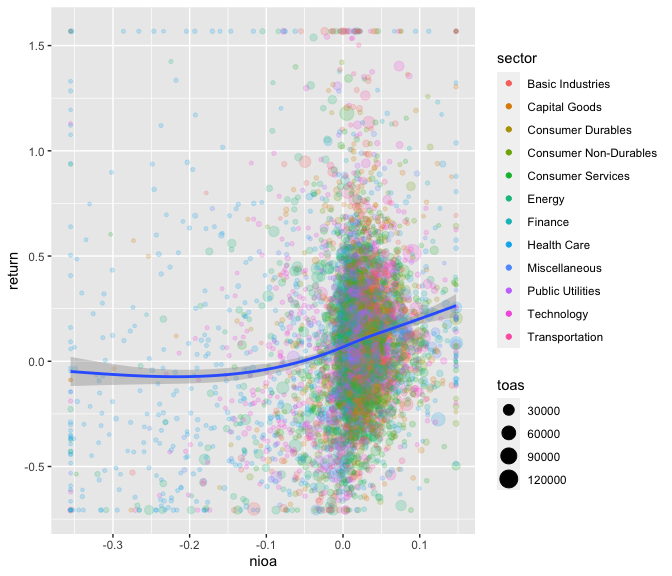Do you see the structural break around nioa == 0? Researchers in the area of accounting tend to like that kind of stuff.

## Regression Tables

Finally, if you happen to be a fan of starred numbers, you can also quickly produce regression tables by using the function prepare_regression_table() that calls lfe::felm() for OLS and glm() for binary logit models. The tables are then constructed by calling stargazer::stargazer(), allowing for plain text, html and latex output.

You can construct tables by mixing different models…

dvs <- c("return", "return", "return", "return", "return", "return")
idvs <- list(c("nioa"),
c("cfoa"),
c("accoa"),
c("cfoa", "accoa"),
c("nioa", "accoa"),
c("nioa", "accoa"))
feffects <- list("period", "period", "period",
c("coid", "period"), c("coid", "period"), c("coid", "period"))
clusters <- list("", "", "", "coid", "coid", c("coid", "period"))
t <- prepare_regression_table(r3win, dvs, idvs, feffects, clusters)
htmltools::HTML(t$table)  Dependent variable: return return return return return return (1) (2) (3) (4) (5) (6) nioa 0.772*** 1.794*** 1.794*** (0.064) (0.305) (0.406) cfoa 0.705*** 1.582*** (0.073) (0.326) accoa 0.534*** 0.876*** -0.890*** -0.890** (0.116) (0.303) (0.314) (0.439) Estimator ols ols ols ols ols ols Fixed effects period period period coid, period coid, period coid, period Std. errors clustered No No No coid coid coid, period Observations 6,002 6,002 6,002 6,002 6,002 6,002 R2 0.023 0.015 0.004 0.013 0.018 0.018 Adjusted R2 0.023 0.015 0.003 -0.564 -0.556 -0.556 Note: *p<0.1; **p<0.05; ***p<0.01 … or by applying one model on different sub-samples. t <- prepare_regression_table(r3win, "return", c("nioa", "accoa"), byvar="period") htmltools::HTML(t$table)
 Dependent variable: return Full Sample FY2014 FY2015 FY2016 (1) (2) (3) (4) nioa 0.801*** 0.234* 0.607*** 1.375*** (0.074) (0.139) (0.123) (0.119) accoa -0.067 0.014 0.638*** -1.224*** (0.132) (0.240) (0.205) (0.233) Constant 0.082*** 0.130*** 0.014 0.096*** (0.006) (0.011) (0.011) (0.011) Estimator ols ols ols ols Fixed effects None None None None Std. errors clustered No No No No Observations 6,002 1,817 2,033 2,152 R2 0.023 0.002 0.033 0.058 Adjusted R2 0.023 0.001 0.032 0.057 Note: *p<0.1; **p<0.05; ***p<0.01

## Conclusion

This is all there is (currently). All these functions are rather simple wrappers around established R functions. They can easily be modified to fit your needs and taste. Take look at the github repository of the ExPanDaR package for the code. Have fun!